## Three vertices of a parallelogram are shown in the figure below. Give the coordinates of the fourth vertex. (-3,9) (0,-3)

Question

Three vertices of a parallelogram are shown in the figure below.
Give the coordinates of the fourth vertex.
(-3,9)
(0,-3) (6,-6)

in progress 0
2 weeks 2021-09-04T11:18:58+00:00 1 Answers 0 views 0

1. Given:

The three vertices of the parallelogram are (-3,9), (0,-3), (6,-6).

To find:

The fourth vertex of the parallelogram.

Solution:

Consider the three vertices of the parallelogram are A(-3,9), B(0,-3), C(6,-6).

Let D(a,b) be the fourth vertex.

Midpoint formula: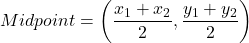We know that the diagonals of a parallelogram bisect each other. So, the midpoints of both diagonals are same.

Midpoint of AC = Midpoint BD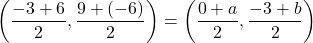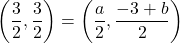On comparing both sides, we get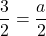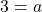And,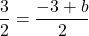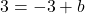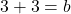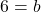Therefore, the fourth vertex of the parallelogram is (3,6).